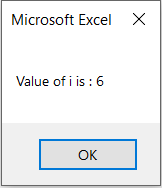# VBA Do Until Loop

The Do Until loop is a useful Excel VBA function that repeats a series of actions until the statement is FALSE. When the statement is FALSE, we want the loop to end and the loop to naturally end.

Also, the condition is specified at the end of the loop, then the Do until Loops condition is checked with each loop iteration, and a decision is made as to whether the loop is true or false.

‘Do Until’ loops are very much like the ‘Do While’ loops.

### Do Until… Loop Statements

Following is the syntax of a Do..Until loop in Excel VBA.

```Do Until condition

[statement 1]

[statement 2]

...

[Exit Do]

[statement 1]

[statement 2]

...

Loop```

### Flow Diagram### Example

Suppose you want to show a MsgBox with the first ten integers using VBA’s Do Until loop.

For that, you must run the loop until the next number is less or equal to 10. A loop would be stopped when the number exceeded 10.

Here is the VBA code to run this loop and display the result in a message box.

```Sub VBADoUntilLoop_Example1()

Dim i As Integer

i = 1

Do Until i > 10

MsgBox i

i = i + 1

Loop

End Sub```

The above loop continues to work until the value of ‘i’ becomes 11. As soon as it becomes 11, the loop ends (as the ‘Until’ condition becomes True).

### Do… Until Loop Statements

Do…Until loop is used to check the condition at the end of the loop.

### Syntax

```Do

[statement 1]

[statement 2]

...

[Exit Do]

[statement 1]

[statement 2]

...

Loop Until condition```

### Flow Diagram### Example

The following example uses the Do…Until loop to check the condition at the end of the loop. Even if the condition is True, all statements within the loop are executed at least once.

```Sub VBADoUntilLoop_Example2()

Dim i As Integer

i = 0

Do

i = i + 1

Loop Until i > 5

MsgBox "Value of i is : " & i

End Sub```

After executing the code, you will get the following output in a message box.## Exit Do Statement

You can exit the loop by using the ‘Exit Do’ statement: as soon as the code executes the ‘Exit Do’ line, it exits the Do Until loop and passes control to the next line immediately after.

For example, you want to show a MsgBox with the first 5 numbers only, then you can exit the loop as soon as the first 5 numbers are entered, as shown in the code below:

```Sub VBADoUntilLoop_Example3()

Dim i As Integer

i = 1

Do Until i > 10

If i = 5 Then Exit Do

MsgBox i

i = i + 1

Loop

End Sub```

In the above code, as soon as the value of ‘i’ becomes 5, Exit Do statment is executed and the loop ends.

Scroll to Top